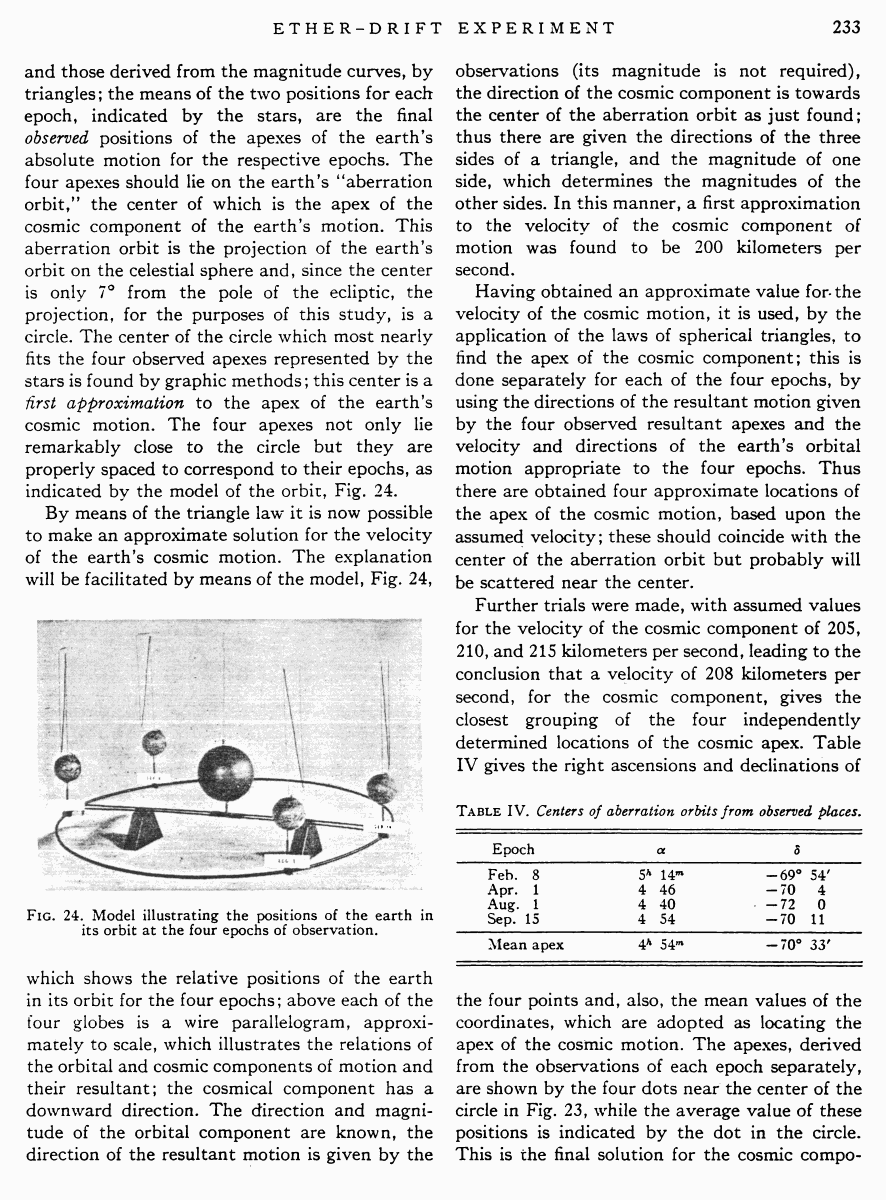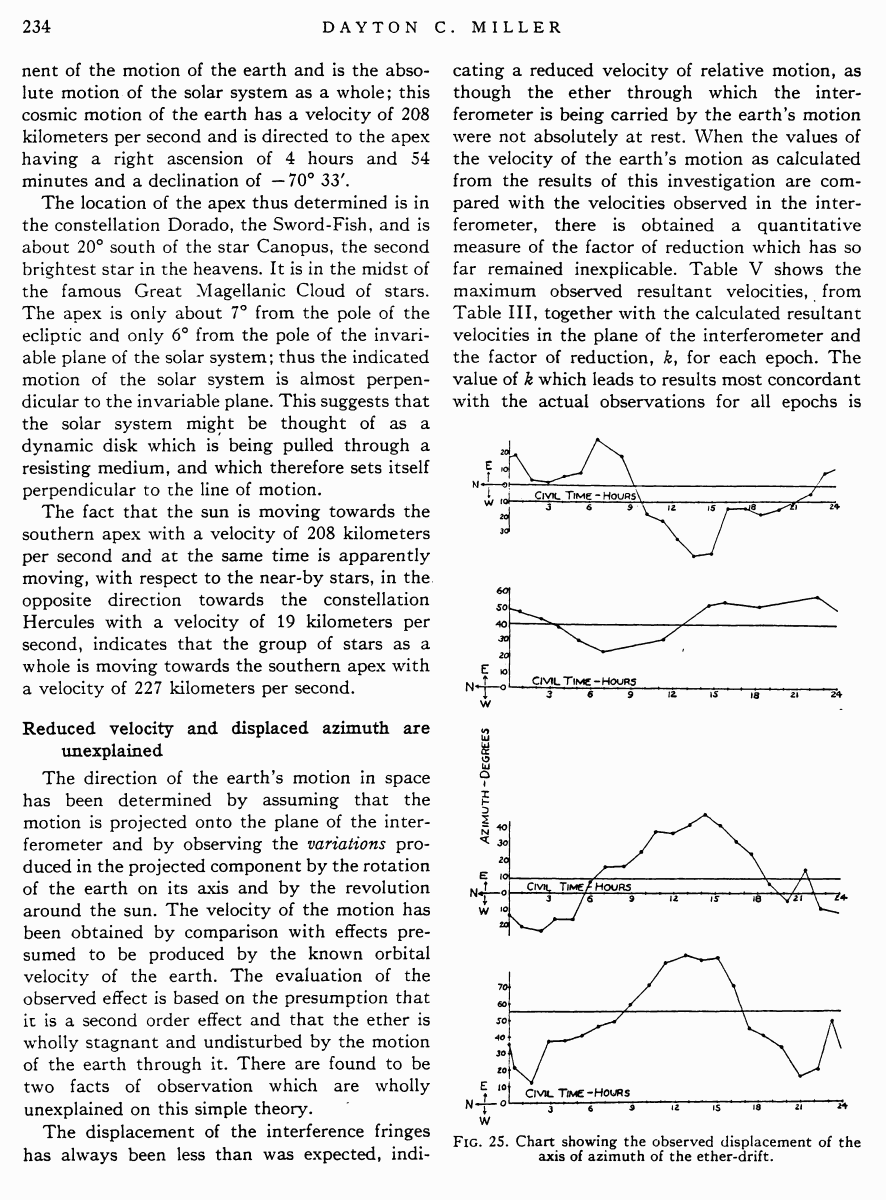# Miller D.C. The Ether-Drift Experiment and the Determination of the Absolute Motion of the Earth // Reviews of modern physics, Vol.5, July 1933

Start   PDF   <<<  Page 233   >>>203  204  205  206  207  208  209  210  211  212  213  214  215  216  217  218  219  220  221  222  223  224  225  226  227  228  229  230  231  232  233 234  235  236  237  238  239  240  241  242

and those derived from the magnitude curves, by triangles; the means of the two positions for each epoch, indicated by the stars, are the final observed positions of the apexes of the earthÆs absolute motion for the respective epochs. The four apexes should lie on the earthÆs ōaberration orbit,ö the center of which is the apex of the cosmic component of the earthÆs motion. This aberration orbit is the projection of the earthÆs orbit on the celestial sphere and, since the center is only 7░ from the pole of the ecliptic, the projection, for the purposes of this study, is a circle. The center of the circle which most nearly fits the four observed apexes represented by the stars is found by graphic methods; this center is a first approximation to the apex of the earthÆs cosmic motion. The four apexes not only lie remarkably close to the circle but they are properly spaced to correspond to their epochs, as indicated by the model of the orbit, Fig. 24.

By means of the triangle law it is now possible to make an approximate solution for the velocity of the earthÆs cosmic motion. The explanation will be facilitated by means of the model, Fig. 24,

Fig. 24. Model illustrating the positions of the earth in its orbit at the four epochs of observation.

which shows the relative positions of the earth in its orbit for the four epochs; above each of the four globes is a wire parallelogram, approximately to scale, which illustrates the relations of the orbital and cosmic components of motion and their resultant; the cosmical component has a downward direction. The direction and magnitude of the orbital component are known, the direction of the resultant motion is given by the

observations (its magnitude is not required), the direction of the cosmic component is towards the center of the aberration orbit as just found; thus there are given the directions of the three sides of a triangle, and the magnitude of one side, which determines the magnitudes of the other sides. In this manner, a first approximation to the velocity of the cosmic component of motion was found to be 200 kilometers per second.

Having obtained an approximate value for the velocity of the cosmic motion, it is used, by the application of the laws of spherical triangles, to find the apex of the cosmic component; this is done separately for each of the four epochs, by using the directions of the resultant motion given by the four observed resultant apexes and the velocity and directions of the earthÆs orbital motion appropriate to the four epochs. Thus there are obtained four approximate locations of the apex of the cosmic motion, based upon the assumed velocity; these should coincide with the center of the aberration orbit but probably will be scattered near the center.

Further trials were made, with assumed values for the velocity of the cosmic component of 205, 210, and 215 kilometers per second, leading to the conclusion that a velocity of 208 kilometers per second, for the cosmic component, gives the closest grouping of the four independently determined locations of the cosmic apex. Table IV gives the right ascensions and declinations of

Table IV. Centers of aberration orbits from observed places.

 Epoch α δ Feb. 8 5h 14m ¢69░ 54′ Apr. 1 4 46 ¢70 4 Aug. 1 4 40 ¢72 0 Sep. 15 4 54 ¢70 11 Mean apex 4h 54m ¢70░ 33′

the four points and, also, the mean values of the coordinates, which are adopted as locating the apex of the cosmic motion. The apexes, derived from the observations of each epoch separately, are shown by the four dots near the center of the circle in Fig. 23, while the average value of these positions is indicated by the dot in the circle. This is the final solution for the cosmic compo

nent of the motion of the earth and is the absolute motion of the solar system as a whole; this cosmic motion of the earth has a velocity of 208 kilometers per second and is directed to the apex having a right ascension of 4 hours and 54 minutes and a declination of ¢70░ 33′.

The location of the apex thus determined is in the constellation Dorado, the Sword-Fish, and is about 20░ south of the star Canopus, the second brightest star in the heavens. It is in the midst of the famous Great Magellanic Cloud of stars. The apex is only about 7░ from the pole of the ecliptic and only 6░ from the pole of the invariable plane of the solar system; thus the indicated motion of the solar system is almost perpendicular to the invariable plane. This suggests that the solar system might be thought of as a dynamic disk which is being pulled through a resisting medium, and which therefore sets itself perpendicular to the line of motion.

The fact that the sun is moving towards the southern apex with a velocity of 208 kilometers per second and at the same time is apparently moving, with respect to the near-by stars, in the opposite direction towards the constellation Hercules with a velocity of 19 kilometers per second, indicates that the group of stars as a whole is moving towards the southern apex with a velocity of 227 kilometers per second.

Reduced velocity and displaced azimuth are unexplained

The direction of the earthÆs motion in space has been determined by assuming that the motion is projected onto the plane of the interferometer and by observing the variations produced in the projected component by the rotation of the earth on its axis and by the revolution around the sun. The velocity of the motion has been obtained by comparison with effects presumed to be produced by the known orbital velocity of the earth. The evaluation of the observed effect is based on the presumption that it is a second order effect and that the ether is wholly stagnant and undisturbed by the motion of the earth through it. There are found to be two facts of observation which are wholly unexplained on this simple theory.

The displacement of the interference fringes has always been less than was expected, indi

cating a reduced velocity of relative motion, as though the ether through which the interferometer is being carried by the earthÆs motion were not absolutely at rest. When the values of the velocity of the earthÆs motion as calculated from the results of this investigation are compared with the velocities observed in the interferometer, there is obtained a quantitative measure of the factor of reduction which has so far remained inexplicable. Table V shows the maximum observed resultant velocities, from Table III, together with the calculated resultant velocities in the plane of the interferometer and the factor of reduction, k, for each epoch. The value of k which leads to results most concordant with the actual observations for all epochs is

Fig. 25. Chart showing the observed displacement of the axis of azimuth of the ether-drift.